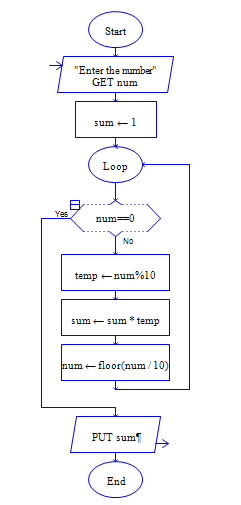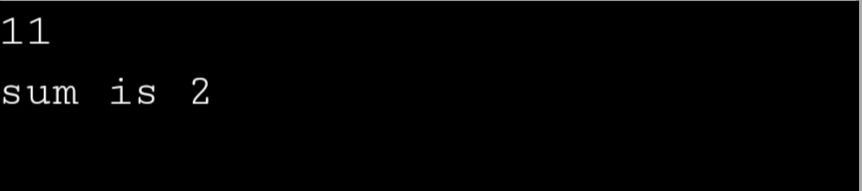# C Program to calculate product of digits.

Sample Input 1 :

734

Sample Output 1 :

84 (7*3*4)

#### Flow Chart Design#### Program or Solution

``` #include<stdio.h> int main() { int num,sum; scanf("%d",&num); while(num!=0) { sum+=num%10; num/=10; } printf("sum is %d",sum); return 0; } ```

#### Output#### Program Explanation

refer video tutorial